Scattering and tunnelling

This free course is available to start right now. Review the full course description and key learning outcomes and create an account and enrol if you want a free statement of participation.

Free course

5.2 Alpha decay

You have probably met the law of radioactive decay, which says that, given a sample of N0 similar nuclei at time t = 0, the number remaining at time t is N(t) = N0eλt, where λ, the decay constant for a particular kind of nucleus, determines the rate at which the nuclei decay. The half-life is the time needed for half of any sufficiently large sample to decay. It is related to the decay constant by T1/2 = (ln2)/λ.

We shall now consider an important type of radioactive decay called alpha decay in which an atomic nucleus emits an energetic alpha particle. The emitted alpha particle consists of two protons and two neutrons and is structurally identical to a helium-4 nucleus (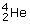). Alpha decay is the dominant decay mode for a number of heavy nuclei (typically those with atomic numbers greater than 84); a specific example is the decay of uranium to thorium represented by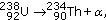where α denotes the alpha particle. Note that the atomic number of the parent nucleus decreases by two and its mass number decreases by four.

Alpha decay was discovered and named by Rutherford in 1898. It was soon established that each type of alpha-decaying nucleus emits an alpha particle with a characteristic energy, Eα. While these alpha emission energies cover a fairly narrow range of values (from about 2 MeV to 8 MeV), the half-lives of the corresponding nuclei cover an enormous range (from 10−12 s to 1017 s). Experiments showed that, within certain families of alpha-emitting nuclei, the half-lives and alpha emission energies were related to one another. Written in terms of the decay constant, λ = (ln 2)/T1/2, this relationship can be expressed in the form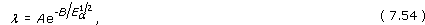where A is a constant that characterises the particular family of nuclei, and B depends on the charge of the individual nucleus. We shall refer to this empirical law as the Geiger-Nuttall relation.

Despite all this information, by the early 1920s alpha decay had become a major puzzle to physicists. The cause of the observed Geiger-Nuttall relation was not understood. Attempts to explain it on the basis of classical physics, with the alpha particle initially confined within the nucleus by an energy barrier that it eventually manages to surmount, did not work. In some cases the observed emission energies were too low to be consistent with surmounting the energy barrier at all. So, how could the alpha particles escape, why did their emission lead to such a staggering range of half-lives, and what was the origin of the Geiger-Nuttall relation?

Answering these questions was one of the early triumphs of wave mechanics. In 1928 the Russian-American physicist George Gamow, and then, independently, Gurney and Condon, proposed a successful theory of alpha decay based on quantum tunnelling. In a simplified version of their approach, the potential energy function responsible for alpha decay has the form shown in Figure 23.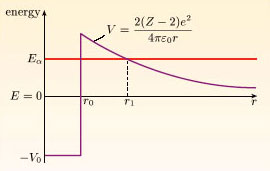Figure 23 The potential energy function V(r) for an alpha particle in the vicinity of an atomic nucleus of atomic number Z

Note that V(r) is a function of a radial coordinate r; this is because we are dealing with a three-dimensional problem in which the potential energy function is spherically symmetric, and r represents the distance from an origin at the centre of the nucleus. Initially, an alpha particle of energy Eα is confined within a distance r = r0 of the origin by the well-like part of the potential energy function. This well is due to the powerful but short-range interaction known as the strong nuclear force. In addition, a long-range electrostatic force acts between the positively charged alpha particle and the remainder of the positively charged nucleus, and has the effect of repelling the alpha particle from the nucleus. The electrostatic force corresponds to the potential energy function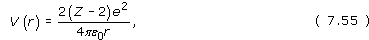where Z is the atomic number of the nucleus, 2e is the charge of the alpha particle, (Z − 2)e is the charge of the nucleus left behind after the decay and ε0 is a fundamental constant called the permittivity of free space. This potential energy function is often called the Coulomb barrier. Notice that the Coulomb barrier exceeds the energy of the alpha particle in the region between r = r0 and r = r1 (defined by V(r1) = Eα). In classical physics, the alpha particle does not have enough energy to enter this region, but in quantum physics it may tunnel through. Once beyond the point r = r1, the alpha particle is electrostatically repelled from the nucleus.

To apply the quantum-mechanical theory of tunnelling to alpha decay, we first note that a classically confined particle would oscillate back and forth inside the well; the combination of its energy (Eα) and the nuclear diameter (2r0) implying that it is incident on the barrier about 1021 times per second. Taking this idea over into quantum mechanics, we shall regard each of these encounters as an escape attempt. The small probability of escape at each attempt is represented by the transmission coefficient for tunnelling, T. To estimate T we must take account of the precise shape of the Coulomb barrier. We shall not go through the detailed arguments used to estimate T in this case, but we shall note that they involve the approximation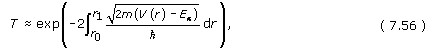where r0 and r1 are the minimum and maximum values of r for which V(r) > Eα. Equation 7.56 is closely related to the expression for tunnelling through a finite square barrier given in Section 4.4. If the potential energy function V(r) happened to be constant over a region of length L, then Equation 7.56 would reproduce the exponential term of Equation 7.53. The other factors in Equation 7.53 are not reproduced, but they vary so slowly compared with the exponential factor that they can be ignored for present purposes.

For given values of Eα, Z and r0, Equation 7.56 can be evaluated using the Coulomb barrier potential energy function of Equation 7.55. After a lengthy calculation, including some approximations, the final result is of the form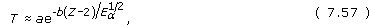where a and b are constants. Multiplying T by the number of escape attempts per second gives the rate of successful escape attempts, and this can be equated to the decay constant, λ. So, according to the quantum tunnelling theory of alpha decay, we have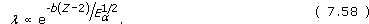This agrees with the Geiger–Nuttall relation and a detailed comparison with experimental data is shown in Figure 24. The exponential-dependence on Eα−1/2 implies that a very wide range of decay constants is associated with a small range of emission energies. The sensitivity to energy is far greater than for a square barrier because of the shape of the Coulomb barrier; increasing the energy of the alpha particle decreases the effective width that must be tunnelled through.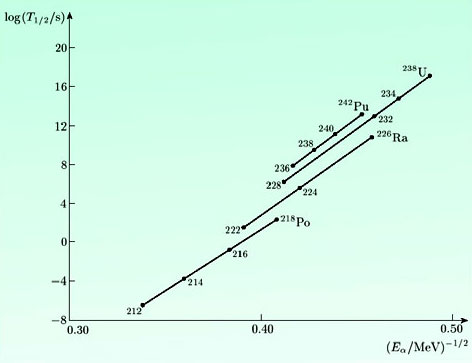Figure 24 A comparison of the Geiger–Nuttall relation with experimental data for different families of nuclei. In this plot, the straight lines confirm the exponential dependence of T1/2 (and hence λ) on Eα−1/2
SM358_1